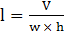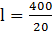### Sample Problem

The volume of a water tank is given by the formula V =. Find the length of the tank if V = 400, w = 5, and h = 4.

l =

#### Solution

V =If V = 400, w = 5, and h = 4.= 20